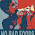### ( wait for it......

I know. Me too.

So, as a public service to help you with your day: )))))))))))))))))))))))))))))))))))))))))))))))))))))))))))))))))))))))))))))))))))))))). That should hold you for at least a day or so, right? You're welcome. ;)

1.Like! :D

2.Hah! Actually I had so much tension yesterday working on income tax returns for H & I and our son (who is away at college), that I can easily deal with punctuation errors today. Now tomorrow, who knows!

3.Too funny and so freaking true! My OCD made me an awesome copy editor.

Here's one of my favorite grammar blog posts:

http://hyperboleandahalf.blogspot.com/2010/04/alot-is-better-than-you-at-everything.html

I also like the Oatmeal's instructions on how to use a semicolon.

4.Epic blog post!

5.I saw this yesterday and it's STILL bothering me.

Malia

6.Any chance you could put another blog up now? This has driven me crazy for TWO DAYS. Please please please do something.

xx